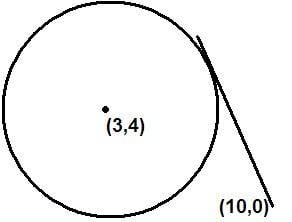# Circle Geometry with tangent

• thomas49th
Thanks everyone for trying though. This question is wierd. :)The wording is fine. The problem simply asks you find the lengths of the two tangent lines(where one of their endpoints is (10,0)) The tangent lines are not going to infinity if that's what you're wondering.There's nothing wrong with the question. It's just asking for the length of the segment of a tangent that starts at (10,0) and ends at the point tangency. From basic geometry, there are two different tangent lines so you have to find the length of the two different segments.Remember that the tangent is perpendicular to the radius. Draw a line from

## Homework Statement

I have the circle of equation (x-3)² + (y-4)² = 5²
Find the exact length of the tangents from the point (10,0) to the circle

## The Attempt at a Solution

Honestly I can't acutally visualise on a co-oridinate graph what it's asking. A tangent touches the circle at the edge, meeting the radius at a right angle. But what does it mean find the exact length of the tangets??

Thanks

If you can't visualize it, then draw it :) You know that the center is at (3,4) and the radius is 5. Now, draw a point at (10,0)

Then ask what is a tangent and how many tangents can there be? Draw the tangents.

Finding the exact lengths of the tangents means exactly what it means. Find the distance from the point that the tangent(s) touches the circle to the point (10,0). Use the distance formula.

The length of the line from point ( 10, 0 ) to the co-ordinate ( x,y) at the point of contact to the circle.

Attempt for the solution

#### Attachments

• CCCCCCCCCCCc.zip
18 KB · Views: 175

thomas49th said:
Honestly I can't acutally visualise on a co-oridinate graph what it's asking. A tangent touches the circle at the edge, meeting the radius at a right angle. But what does it mean find the exact length of the tangets??

Hi thomas49th!m_s_a has given a very nice picture of the co-ordinate graph.

But I would find the distance from (10,0) to the centre of the circle, and then use Pythaogras' theorem (twice).err image had loads of all kinds of stuff i didn't need right? I'm still not sure what I am actually finding out. I can see why you use pythagerous make a right angle triangle out of x and y and find the line using a² + b² = c². That's easy... i just don't know what the question is asking

Here is my diagramBut i don't really have much of an idea what I am meant to be finding.

Just the wording of the question is so, so stupid.

Thanks everyone for trying though. This question is wierd. :)

The wording is fine. The problem simply asks you find the lengths of the two tangent lines(where one of their endpoints is (10,0))

The tangent lines are not going to infinity if that's what you're wondering.

There's nothing wrong with the question. It's just asking for the length of the segment of a tangent that starts at (10,0) and ends at the point tangency. From basic geometry, there are two different tangent lines so you have to find the length of the two different segments.

Remember that the tangent is perpendicular to the radius. Draw a line from (4, 5) to the point of tangency and the line from (4,5) to (10,0) in your picture and you have a right triangle with the line from (4,5) to (10,0) as hypotenuse. You know the length of the radius from (4,5) to the point of tangency and you can calculate the length of the hypotenuse from (4,5) to (10,0). As tiny tim said, use the Pythagorean theorem to find the length of the tangent segment.

do you mean (4,5) or (3,4)?